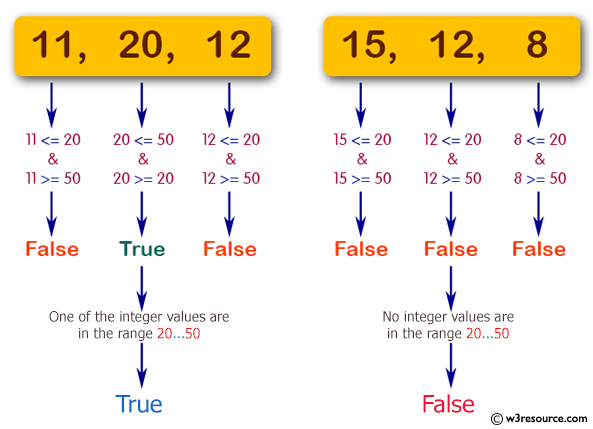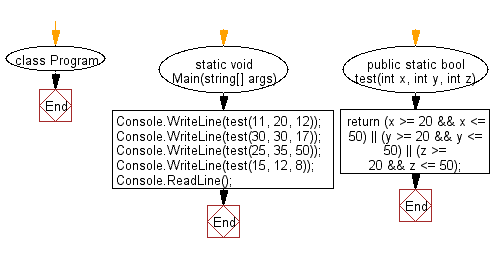﻿ C# - Check whether 3 integer values are between 20 and 50# C# Sharp Basic Algorithm Exercises: Check whether three given integer values are in the range 20..50 inclusive

## C# Sharp Basic Algorithm: Exercise-15 with Solution

Write a C# Sharp program to check whether three given integer values are in the range 20..50 inclusive. Return true if 1 or more of them are in the said range otherwise false.

Pictorial Presentation:Sample Solution:

C# Sharp Code:

``````using System;

namespace exercises
{
class Program
{
static void Main(string[] args)
{
Console.WriteLine(test(11, 20, 12));
Console.WriteLine(test(30, 30, 17));
Console.WriteLine(test(25, 35, 50));
Console.WriteLine(test(15, 12, 8));
}

public static bool test(int x, int y, int z)
{
return (x >= 20 && x <= 50) || (y >= 20 && y <= 50) || (z >= 20 && z <= 50);
}
}
}
```
```

Sample Output:

```True
True
True
False```

Flowchart:C# Sharp Code Editor:

Improve this sample solution and post your code through Disqus

What is the difficulty level of this exercise?

Test your Programming skills with w3resource's quiz.

﻿